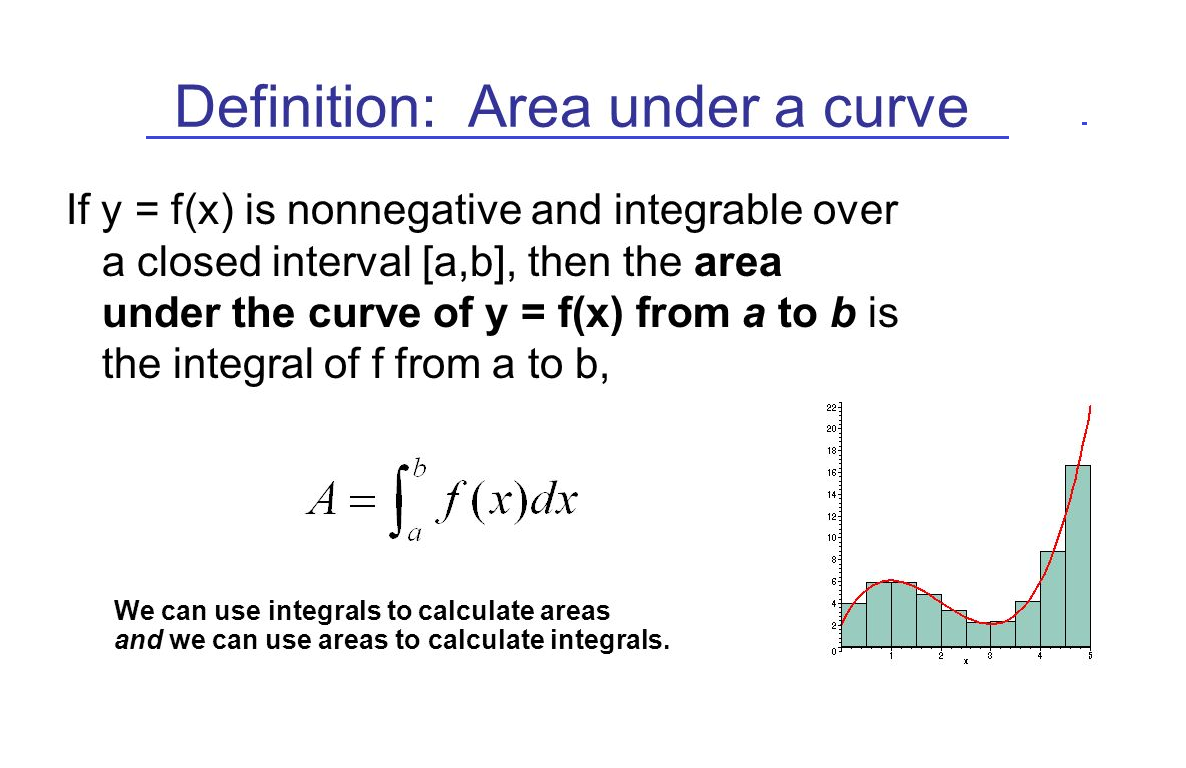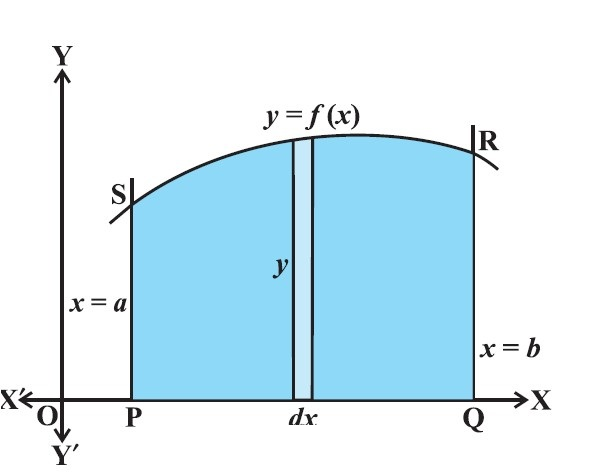Mathematics Quick Revision

### Area under Simple Curves● Total area A of the region between x-axis, ordinates x = a, x = b and the curve y = f (x) as the result of adding up the elementary areas of thin strips across the region PQRSP. Symbolically, we express● Here, we consider vertical strips as shown in the Fig

color{blue} {A = int_a^b d A = int_a^b ydx = int_a^b f(x) dx}

### Area between Two CurvesA = ["area bounded by" y = f (x), "x-axis and the lines"\ \ x = a, x = b]
– ["area bounded by" y = g (x), "x-axis and the lines" \ \ x = a, x = b]

color {red} {= int_a^b f(x) dx - int_a^b g(x) dx = int_a^b [ f(x) - g(x) ] dx} , where f(x) >= g(x)  in [ a,b]